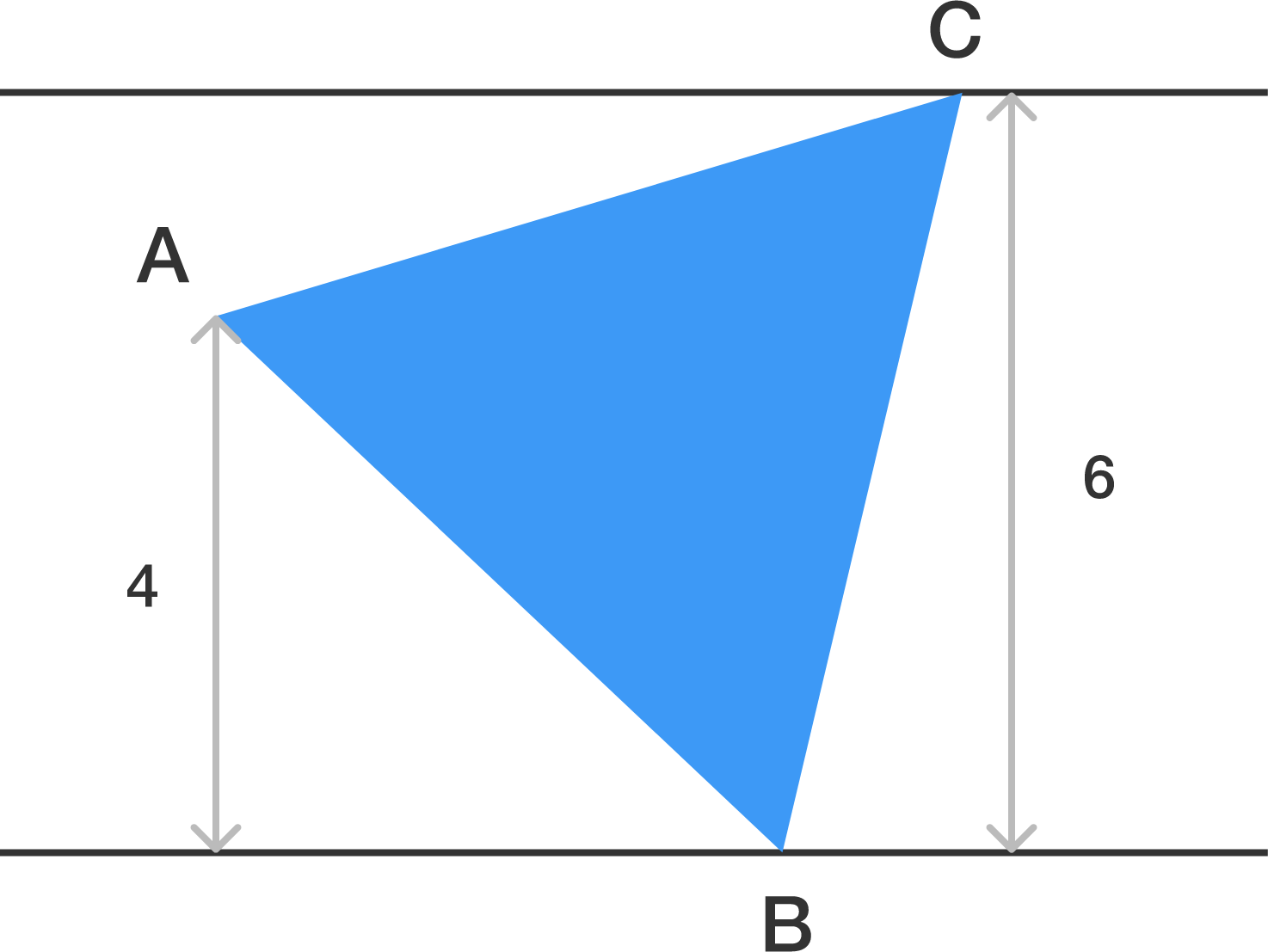Just be quick with calculations

Geometry Level 3

$ABC$ is an equilateral triangle such that vertices $B,C$ lie on two parallel lines at a distance of 6 units. If $A$ lies between the parallel lines at a distance 4 units from one of them, then the length of the side of the triangle is of the form

$\large{A\sqrt{\frac{B}{C}}}$, where $A,B,C$ are co prime natural numbers.

Find $A+B+C$.×